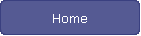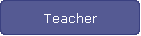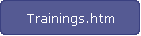Factors

Product Game a two-player game to practice finding factors of numbers.

Factor Trees  Find the prime factorization of numbers by completing factor trees. Then use a Venn diagram to sort the prime factors. Multiply numbers in the intersection to find the greatest common factor.  Multiply all the numbers in the Venn diagram to find the least common multiple.

Factor Game exercises your factoring ability. You can play against the computer or against a friend.

Calculation Nation Click on Guest Pass and then click on Factor Dazzle.

Snake Click on speed and then click on factors, multiples, primes or challenges.  (Pac-Man style game)

The Factor Tree Click on Start Factoring.

Multiplication Station Scroll down and click on Click to Play. Click on the squares to multiply the numbers so they equal the value under the word "Number". If you multiply the numbers fast enough you earn bonus points.  If you click on the wrong numbers, you will have to start over again.

Grid Game Find the factors, multiples, powers, primes and triangular numbers from the selection shown on the grid and see Pythagoras and Hypatia jump for joy!

Created by Linda DeVore

Last Updated Sunday, February 27, 2011

www.librarianscorner.net